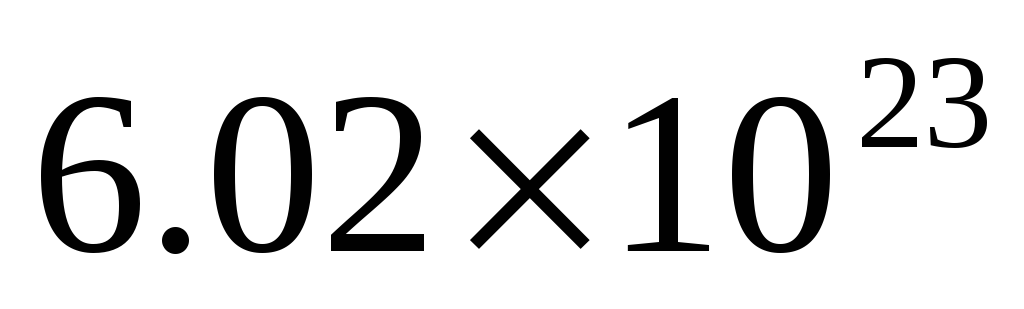# Chemistry Science Curriculum Framework

 Page 3/10 Date 23.04.2018 Size 1.01 Mb. #45990

#### Strand: Bonding

Standard 9: Students shall understand the process of covalent bonding.
 B.9.C.1 Draw Lewis structures to show valence electrons for covalent bonding: lone pairs shared pairs hybridization resonance B.9.C.2 Determine the properties of covalent compounds based upon double and triple bonding B.9.C.3 Predict the polarity and geometry of a molecule based upon shared electron pairs and lone electron pairs: VSEPR Model B.9.C.4 Identify the strengths and effects of intermolecular forces (van der Waals): hydrogen bonding dipole-dipole dipole-induced dipole dispersion forces (London)
##### Strand: Bonding

Standard 10: Students shall understand the process of metallic bonding.
 B.10.C.1 Explain the properties of metals due to delocalized electrons: molecular orbital model
##### Strand: Bonding

Standard 11: Students shall relate the physical properties of solids to different types of bonding.
 B.11.C.1 Distinguish between amorphous and crystalline solids B.11.C.2 Compare and contrast the properties of crystalline solids: ionic covalent network covalent molecular metallic
##### Strand: Stoichiometry

Standard 12: Students shall understand the relationships between balanced chemical equations and mole relationships.
 S.12.C.1 Balance chemical equations when all reactants and products are given S.12.C.2 Use balanced reaction equations to obtain information about the amounts of reactants and products S.12.C.3 Distinguish between limiting reactants and excess reactants in balanced reaction equations S.12.C.4 Calculate stoichiometric quantities and use these to determine theoretical yields
##### Strand: Stoichiometry

Standard 13: Students shall understand the mole concept and Avogadro’s number.
 S.13.C.1 Apply the mole concept to calculate the number of particles and the amount of substance: Avogadro’s constant =S.13.C.2 Determine the empirical and molecular formulas using the molar concept: molar mass average atomic mass molecular mass formula mass
##### Strand: Stoichiometry

Standard 14: Students shall predict products based upon the type of chemical reaction.
 S.14.C.1 Given the reactants predict products for the following types of reactions: synthesis decomposition single displacement double displacement combustion

##### Strand: Stoichiometry

Standard 15: Students shall understand the composition of solutions, their formation, and their strengths expressed in various units.
 S.15.C.1 Distinguish between the terms solute, solvent, solution and concentration S.15.C.2 Give examples for the nine solvent-solute pairs S.15.C.3 Calculate the following concentration expressions involving the amount of solute and volume of solution: molarity (M) molality (m) percent composition normality (N) S.15.C.4 Given the quantity of a solution, determine the quantity of another species in the reaction S.15.C.5 Define heat of solution S.15.C.6 Identify the physical state for each substance in a reaction equation